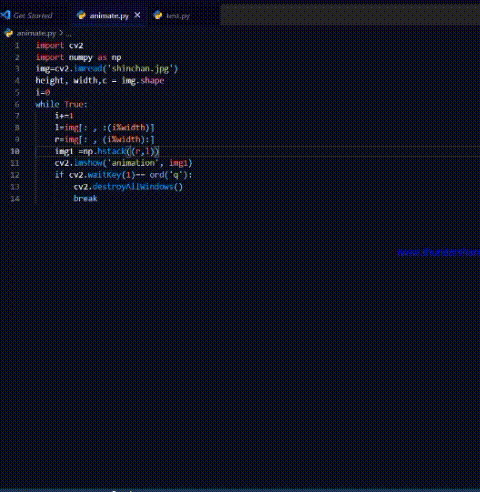# Animate image using OpenCV in Python

• Last Updated : 17 Dec, 2021

In this article, we will discuss how to animate an image using python’s OpenCV module.

Let’s suppose we have one image. Using that single image we will animate it in such a way it will appear continuous array of the same image. This is useful in animating the background in certain games. For example, in a flappy bird game to make the bird appear moving forward the background needs to move backward.  To understand this let us first consider a linear python list. Consider the following code for a moment.

## Python3

 `a ``=` `[``'-'``, ``'-'``, ``'-'``, ``1``, ``'-'``, ``'-'``, ``'-'``]``n ``=` `len``(a)  ``# length of the array` `for` `i ``in` `range``(``2``*``n):``    ``# i is the index of the list a``    ``# i%n will have a value in range(0,n)``    ``# using slicing we can make the digit 1``    ``# appear to move across the list``    ``# this is similar to a cyclic list``    ``print``(a[(i ``%` `n):]``+``a[:(i ``%` `n)])`

Output:

```['-', '-', '-', 1, '-', '-', '-']
['-', '-', 1, '-', '-', '-', '-']
['-', 1, '-', '-', '-', '-', '-']
[1, '-', '-', '-', '-', '-', '-']
['-', '-', '-', '-', '-', '-', 1]
['-', '-', '-', '-', '-', 1, '-']
['-', '-', '-', '-', 1, '-', '-']
['-', '-', '-', 1, '-', '-', '-']
['-', '-', 1, '-', '-', '-', '-']
['-', 1, '-', '-', '-', '-', '-']
[1, '-', '-', '-', '-', '-', '-']
['-', '-', '-', '-', '-', '-', 1]
['-', '-', '-', '-', '-', 1, '-']
['-', '-', '-', '-', 1, '-', '-']```

From the above code, we can see that the position of digit 1 is changing i.e. the index is changing. This is the principle we will use to animate the image horizontally.

We will concatenate the two images using hstack() function from the NumPy module. hstack function takes a tuple consisting of the order of the arrays as arguments and is used to stack the sequence of input arrays horizontally (i.e. column-wise) to make a single array.

Syntax:

`numpy.hstack((array1,array2))`

Example:

## Python3

 `import` `cv2``import` `numpy as np` `img ``=` `cv2.imread(``'shinchan.jpg'``)` `height, width, c ``=` `img.shape` `i ``=` `0` `while` `True``:``    ``i ``+``=` `1``    ` `    ``# divided the image into left and right part``    ``# like list concatenation we concatenated``    ``# right and left together``    ``l ``=` `img[:, :(i ``%` `width)]``    ``r ``=` `img[:, (i ``%` `width):]` `    ``img1 ``=` `np.hstack((r, l))``    ` `    ``# this function will concatenate``    ``# the two matrices``    ``cv2.imshow(``'animation'``, img1)` `    ``if` `cv2.waitKey(``1``) ``=``=` `ord``(``'q'``):``      ` `        ``# press q to terminate the loop``        ``cv2.destroyAllWindows()``        ``break`

Output:My Personal Notes arrow_drop_up